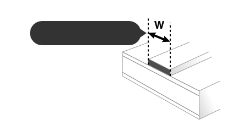# PCB Trace Width Calculator

This tool uses formulas from IPC-2221 to calculate the width of a copper printed circuit board conductor or "trace" required to carry a given current while keeping the resulting increase in trace temperature below a specified limit. If the length of the trace is also provided, the total resistance, voltage drop, and power loss due to trace resistance are also calculated. The results are estimates; actual results may vary depending on application conditions. Note also that required widths are significantly higher for traces on internal layers of a circuit board compared to those on a board’s outer surface; use whichever result is appropriate for your situation.

Current (I)
A
Thickness (t)
Temperature Rise (TRise)
Ambient Temperature
Trace Length

## Formula

First, calculate the Area:

Then, calculate the Width:

For IPC-2221 internal layers: k = 0.024, b = 0.44, c = 0.725

For IPC-2221 external layers: k = 0.048, b = 0.44, c = 0.725

where k, b, and c are constants resulting from curve fitting to the IPC-2221 curves.

Common values:

Thickness: 1 oz

Ambient: 25 C

Temp rise: 10 C

Minimum Trace Width
mil### Internal Layers

Required Trace Width (W)
Resistance
Ω
Voltage Drop
V
Power Loss
W
Minimum Trace Width
mil### External Layers in Air

Required Trace Width (W)
Resistance
Ω
Voltage Drop
V
Power Loss
W